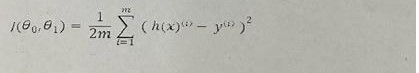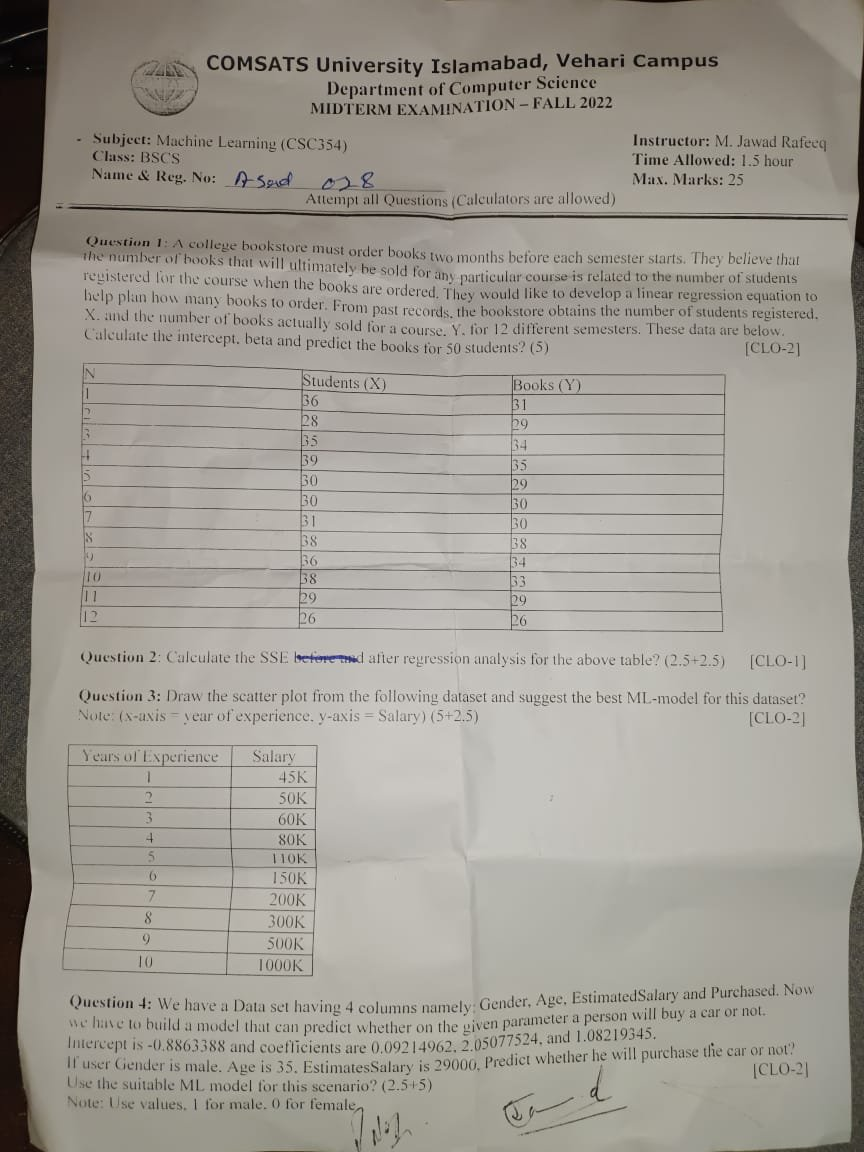# Machine learning all previous/ past question papers

– Machine learning-Computer Science all subject past paper Machine learning –Computer Graphics  Course  Machine learning Project idea – ICT  MCQ -Machine learning Interview Question  –Computer Science all courses -Technology –University Past Paper -Programming language –Question paperold paper

Q1: A model has been tested and the following model evaluation measures are observed. Calculate the MSE and MAE and which one is better for this dataset?

 Y(Actual) Y’(Predicted) 10.2 9.4 3.5 1.7 7.1 6.9 14.5 15.4 17.2 18.4 41.5 17.2 2.7 2.5 11.5 11.1 5.9 6.7 15.3 15.2

Q2: If engine Size = 130, horsepower= 111, PeakRpm = 5000, Length = 16, Width = 6, Height = 13, Calculate the price of car and write the model name which is suitable for this example?

Q3: By using the following cost function of gradient descent, derive the equation for third co-effient?Q4: An OLS model is trained on the dataset and the following results are stated in the table. Calculate the R2 from the following table? What does that R-squared value mean?

 y-actual Y-Predicted 700 782.3275862 900 977.5862069 1300 1172.844828 1750 1563.352069 1800 1953.87931#Machine learning #Computer Science all subject past paper  Machine learning #Computer Graphics  Course  #Machine learning Project idea  # Machine learning MCQ  #Machine learning Interview Question  #Computer Science all courses #Technology #University Past Paper #Programming language  #Question paper  #old paper

Scroll to Top